# 5.3 Graphs of polynomial functions  (Page 9/13)

 Page 9 / 13

$f\left(x\right)={x}^{5}-2x,\text{\hspace{0.17em}}$ between $\text{\hspace{0.17em}}x=1\text{\hspace{0.17em}}$ and $\text{\hspace{0.17em}}x=2.$

$f\left(x\right)=-{x}^{4}+4,\text{\hspace{0.17em}}$ between $\text{\hspace{0.17em}}x=1\text{\hspace{0.17em}}$ and $\text{\hspace{0.17em}}x=3$ .

$f\left(1\right)=3\text{\hspace{0.17em}}$ and $\text{\hspace{0.17em}}f\left(3\right)=–77.\text{\hspace{0.17em}}$ Sign change confirms.

$f\left(x\right)=-2{x}^{3}-x,\text{\hspace{0.17em}}$ between $\text{\hspace{0.17em}}x=–1\text{\hspace{0.17em}}$ and $\text{\hspace{0.17em}}x=1.$

$f\left(x\right)={x}^{3}-100x+2,\text{\hspace{0.17em}}$ between $\text{\hspace{0.17em}}x=0.01\text{\hspace{0.17em}}$ and $\text{\hspace{0.17em}}x=0.1$

$f\left(0.01\right)=1.000001\text{\hspace{0.17em}}$ and $\text{\hspace{0.17em}}f\left(0.1\right)=–7.999.\text{\hspace{0.17em}}$ Sign change confirms.

For the following exercises, find the zeros and give the multiplicity of each.

$f\left(x\right)={\left(x+2\right)}^{3}{\left(x-3\right)}^{2}$

$f\left(x\right)={x}^{2}{\left(2x+3\right)}^{5}{\left(x-4\right)}^{2}$

0 with multiplicity 2, $\text{\hspace{0.17em}}-\frac{3}{2}\text{\hspace{0.17em}}$ with multiplicity 5, 4 with multiplicity 2

$f\left(x\right)={x}^{3}{\left(x-1\right)}^{3}\left(x+2\right)$

$f\left(x\right)={x}^{2}\left({x}^{2}+4x+4\right)$

0 with multiplicity 2, –2 with multiplicity 2

$f\left(x\right)={\left(2x+1\right)}^{3}\left(9{x}^{2}-6x+1\right)$

$f\left(x\right)={\left(3x+2\right)}^{5}\left({x}^{2}-10x+25\right)$

$-\frac{2}{3}\text{\hspace{0.17em}}\text{with}\text{\hspace{0.17em}}\text{multiplicity}\text{\hspace{0.17em}}5\text{,}\text{\hspace{0.17em}}5\text{\hspace{0.17em}}\text{with}\text{\hspace{0.17em}}\text{multiplicity}\text{\hspace{0.17em}}\text{2}$

$f\left(x\right)=x\left(4{x}^{2}-12x+9\right)\left({x}^{2}+8x+16\right)$

$f\left(x\right)={x}^{6}-{x}^{5}-2{x}^{4}$

$\text{0}\text{\hspace{0.17em}}\text{with}\text{\hspace{0.17em}}\text{multiplicity}\text{\hspace{0.17em}}4\text{,}\text{\hspace{0.17em}}2\text{\hspace{0.17em}}\text{with}\text{\hspace{0.17em}}\text{multiplicity}\text{\hspace{0.17em}}1\text{,}\text{\hspace{0.17em}}–\text{1}\text{\hspace{0.17em}}\text{with}\text{\hspace{0.17em}}\text{multiplicity}\text{\hspace{0.17em}}1$

$f\left(x\right)=3{x}^{4}+6{x}^{3}+3{x}^{2}$

$f\left(x\right)=4{x}^{5}-12{x}^{4}+9{x}^{3}$

$\frac{3}{2}\text{\hspace{0.17em}}$ with multiplicity 2, 0 with multiplicity 3

$f\left(x\right)=2{x}^{4}\left({x}^{3}-4{x}^{2}+4x\right)$

$f\left(x\right)=4{x}^{4}\left(9{x}^{4}-12{x}^{3}+4{x}^{2}\right)$

$\text{0}\text{\hspace{0.17em}}\text{with}\text{\hspace{0.17em}}\text{multiplicity}\text{\hspace{0.17em}}6\text{,}\text{\hspace{0.17em}}\frac{2}{3}\text{\hspace{0.17em}}\text{with}\text{\hspace{0.17em}}\text{multiplicity}\text{\hspace{0.17em}}2$

## Graphical

For the following exercises, graph the polynomial functions. Note $\text{\hspace{0.17em}}x\text{-}$ and $\text{\hspace{0.17em}}y\text{-}$ intercepts, multiplicity, and end behavior.

$f\left(x\right)={\left(x+3\right)}^{2}\left(x-2\right)$

$g\left(x\right)=\left(x+4\right){\left(x-1\right)}^{2}$

x -intercepts, $\left(1, 0\right)$ with multiplicity 2, with multiplicity 1, $y\text{-}$ intercept As $\phantom{\rule{0.2em}{0ex}}x\to -\infty ,\phantom{\rule{0.2em}{0ex}}f\left(x\right)\to -\infty ,\text{\hspace{0.17em}}\text{as}\phantom{\rule{0.2em}{0ex}}x\to \infty ,\phantom{\rule{0.2em}{0ex}}f\left(x\right)\to \infty .$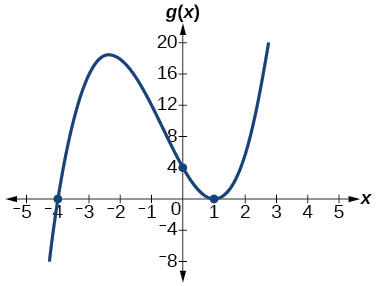$h\left(x\right)={\left(x-1\right)}^{3}{\left(x+3\right)}^{2}$

$k\left(x\right)={\left(x-3\right)}^{3}{\left(x-2\right)}^{2}$

x -intercepts $\text{\hspace{0.17em}}\left(3,0\right)\text{\hspace{0.17em}}$ with multiplicity 3, $\text{\hspace{0.17em}}\left(2,0\right)\text{\hspace{0.17em}}$ with multiplicity 2, $\text{\hspace{0.17em}}y\text{-}$ intercept $\text{\hspace{0.17em}}\left(0,–108\right).\text{\hspace{0.17em}}$ As $x\to -\infty ,\phantom{\rule{0.2em}{0ex}}f\left(x\right)\to -\infty ,\phantom{\rule{0.2em}{0ex}}\text{as}\phantom{\rule{0.2em}{0ex}}x\to \infty ,\phantom{\rule{0.2em}{0ex}}f\left(x\right)\to \infty .$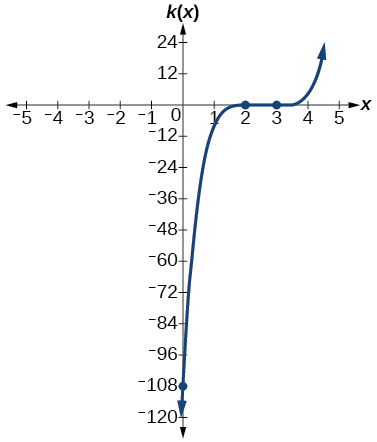$m\left(x\right)=-2x\left(x-1\right)\left(x+3\right)$

$n\left(x\right)=-3x\left(x+2\right)\left(x-4\right)$

x -intercepts with multiplicity 1, $\text{\hspace{0.17em}}y\text{-}$ intercept As $x\to -\infty ,\phantom{\rule{0.2em}{0ex}}f\left(x\right)\to \infty ,\phantom{\rule{0.2em}{0ex}}\text{as}\phantom{\rule{0.2em}{0ex}}x\to \infty ,\phantom{\rule{0.2em}{0ex}}f\left(x\right)\to -\infty .$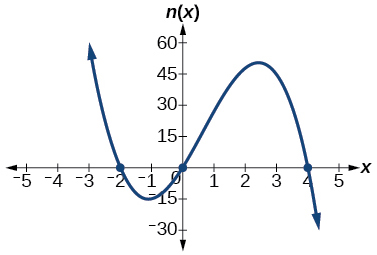For the following exercises, use the graphs to write the formula for a polynomial function of least degree.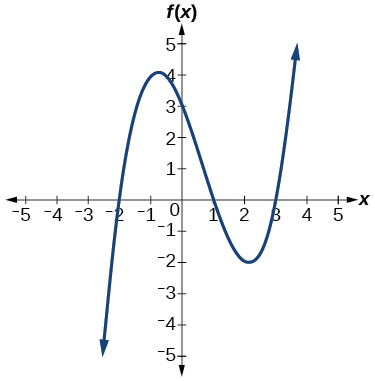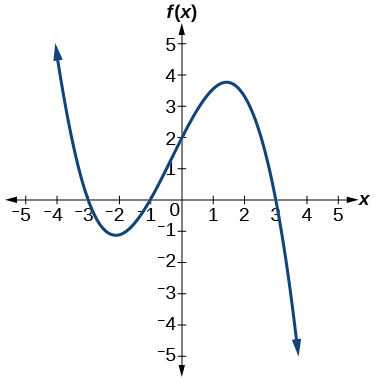$f\left(x\right)=-\frac{2}{9}\left(x-3\right)\left(x+1\right)\left(x+3\right)$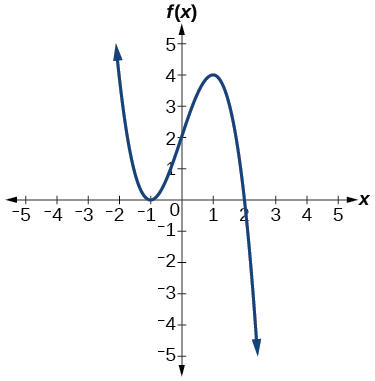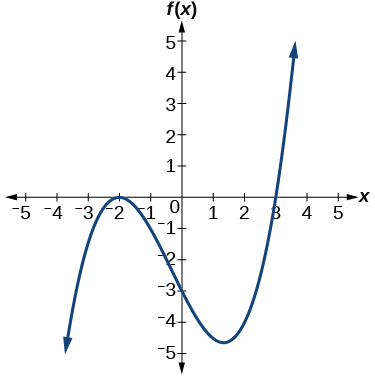$f\left(x\right)=\frac{1}{4}{\left(x+2\right)}^{2}\left(x-3\right)$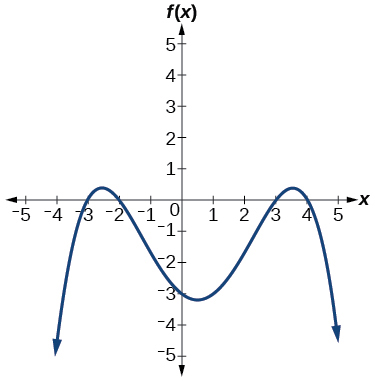For the following exercises, use the graph to identify zeros and multiplicity.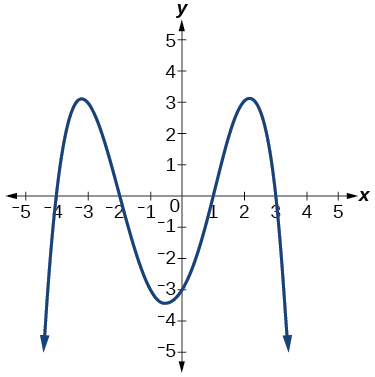–4, –2, 1, 3 with multiplicity 1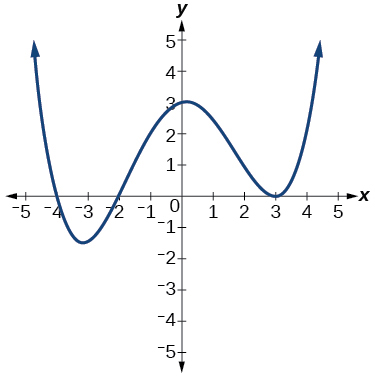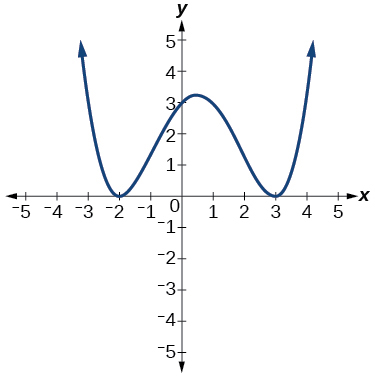–2, 3 each with multiplicity 2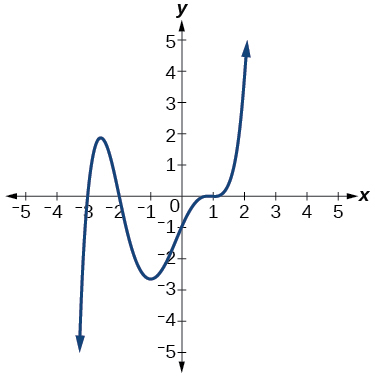For the following exercises, use the given information about the polynomial graph to write the equation.

Degree 3. Zeros at $\text{\hspace{0.17em}}x=–2,$ $\text{\hspace{0.17em}}x=1,\text{\hspace{0.17em}}$ and $\text{\hspace{0.17em}}x=3.\text{\hspace{0.17em}}$ y -intercept at $\text{\hspace{0.17em}}\left(0,–4\right).$

$f\left(x\right)=-\frac{2}{3}\left(x+2\right)\left(x-1\right)\left(x-3\right)$

Degree 3. Zeros at $\text{\hspace{0.17em}}x=\text{–5,}$ $\text{\hspace{0.17em}}x=–2,$ and $\text{\hspace{0.17em}}x=1.\text{\hspace{0.17em}}$ y -intercept at $\text{\hspace{0.17em}}\left(0,6\right)$

Degree 5. Roots of multiplicity 2 at $\text{\hspace{0.17em}}x=3\text{\hspace{0.17em}}$ and $\text{\hspace{0.17em}}x=1\text{\hspace{0.17em}}$ , and a root of multiplicity 1 at $\text{\hspace{0.17em}}x=–3.\text{\hspace{0.17em}}$ y -intercept at $\text{\hspace{0.17em}}\left(0,9\right)$

$f\left(x\right)=\frac{1}{3}{\left(x-3\right)}^{2}{\left(x-1\right)}^{2}\left(x+3\right)$

Degree 4. Root of multiplicity 2 at $\text{\hspace{0.17em}}x=4,\text{\hspace{0.17em}}$ and a roots of multiplicity 1 at $\text{\hspace{0.17em}}x=1\text{\hspace{0.17em}}$ and $\text{\hspace{0.17em}}x=–2.\text{\hspace{0.17em}}$ y -intercept at $\text{\hspace{0.17em}}\left(0,\text{–}3\right).$

Degree 5. Double zero at $\text{\hspace{0.17em}}x=1,\text{\hspace{0.17em}}$ and triple zero at $\text{\hspace{0.17em}}x=3.\text{\hspace{0.17em}}$ Passes through the point $\text{\hspace{0.17em}}\left(2,15\right).$

$f\left(x\right)=-15{\left(x-1\right)}^{2}{\left(x-3\right)}^{3}$

Degree 3. Zeros at $\text{\hspace{0.17em}}x=4,$ $\text{\hspace{0.17em}}x=3,$ and $\text{\hspace{0.17em}}x=2.\text{\hspace{0.17em}}$ y -intercept at $\text{\hspace{0.17em}}\left(0,-24\right).$

Degree 3. Zeros at $\text{\hspace{0.17em}}x=-3,$ $\text{\hspace{0.17em}}x=-2\text{\hspace{0.17em}}$ and $\text{\hspace{0.17em}}x=1.\text{\hspace{0.17em}}$ y -intercept at $\text{\hspace{0.17em}}\left(0,12\right).$

$f\left(x\right)=-2\left(x+3\right)\left(x+2\right)\left(x-1\right)$

Degree 5. Roots of multiplicity 2 at $\text{\hspace{0.17em}}x=-3\text{\hspace{0.17em}}$ and $\text{\hspace{0.17em}}x=2\text{\hspace{0.17em}}$ and a root of multiplicity 1 at $\text{\hspace{0.17em}}x=-2.$

y -intercept at

Degree 4. Roots of multiplicity 2 at $\text{\hspace{0.17em}}x=\frac{1}{2}\text{\hspace{0.17em}}$ and roots of multiplicity 1 at $\text{\hspace{0.17em}}x=6\text{\hspace{0.17em}}$ and $\text{\hspace{0.17em}}x=-2.$

y -intercept at $\text{\hspace{0.17em}}\left(0,18\right).$

$f\left(x\right)=-\frac{3}{2}{\left(2x-1\right)}^{2}\left(x-6\right)\left(x+2\right)$

Double zero at $\text{\hspace{0.17em}}x=-3\text{\hspace{0.17em}}$ and triple zero at $\text{\hspace{0.17em}}x=0.\text{\hspace{0.17em}}$ Passes through the point $\text{\hspace{0.17em}}\left(1,32\right).$

## Technology

For the following exercises, use a calculator to approximate local minima and maxima or the global minimum and maximum.

$f\left(x\right)={x}^{3}-x-1$

local max local min

$f\left(x\right)=2{x}^{3}-3x-1$

$f\left(x\right)={x}^{4}+x$

global min

$f\left(x\right)=-{x}^{4}+3x-2$

$f\left(x\right)={x}^{4}-{x}^{3}+1$

global min

## Extensions

For the following exercises, use the graphs to write a polynomial function of least degree.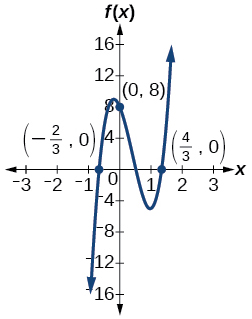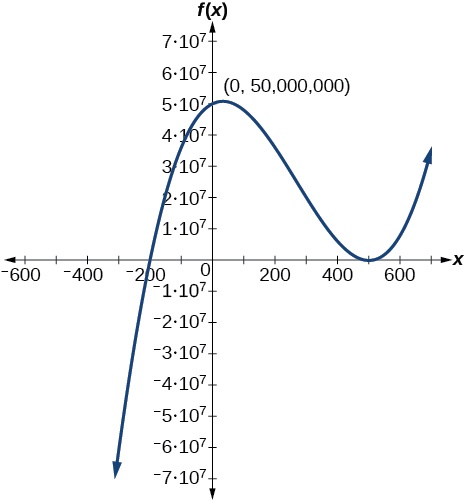$f\left(x\right)={\left(x-500\right)}^{2}\left(x+200\right)$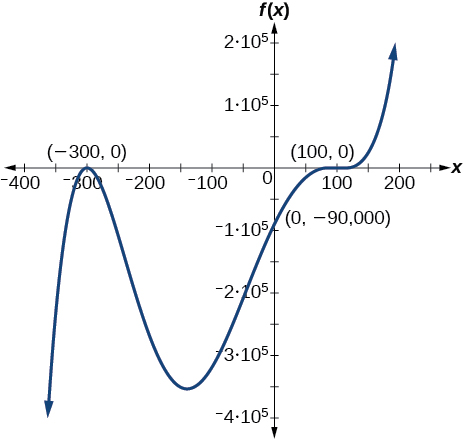## Real-world applications

For the following exercises, write the polynomial function that models the given situation.

A rectangle has a length of 10 units and a width of 8 units. Squares of $\text{\hspace{0.17em}}x\text{\hspace{0.17em}}$ by $\text{\hspace{0.17em}}x\text{\hspace{0.17em}}$ units are cut out of each corner, and then the sides are folded up to create an open box. Express the volume of the box as a polynomial function in terms of $\text{\hspace{0.17em}}x.$

$f\left(x\right)=4{x}^{3}-36{x}^{2}+80x$

Consider the same rectangle of the preceding problem. Squares of $\text{\hspace{0.17em}}2x\text{\hspace{0.17em}}$ by $\text{\hspace{0.17em}}2x\text{\hspace{0.17em}}$ units are cut out of each corner. Express the volume of the box as a polynomial in terms of $\text{\hspace{0.17em}}x.$

A square has sides of 12 units. Squares by units are cut out of each corner, and then the sides are folded up to create an open box. Express the volume of the box as a function in terms of $\text{\hspace{0.17em}}x.$

$f\left(x\right)=4{x}^{3}-36{x}^{2}+60x+100$

A cylinder has a radius of $\text{\hspace{0.17em}}x+2\text{\hspace{0.17em}}$ units and a height of 3 units greater. Express the volume of the cylinder as a polynomial function.

A right circular cone has a radius of $\text{\hspace{0.17em}}3x+6\text{\hspace{0.17em}}$ and a height 3 units less. Express the volume of the cone as a polynomial function. The volume of a cone is $\text{\hspace{0.17em}}V=\frac{1}{3}\pi {r}^{2}h\text{\hspace{0.17em}}$ for radius $\text{\hspace{0.17em}}r\text{\hspace{0.17em}}$ and height $\text{\hspace{0.17em}}h.$

$f\left(x\right)=\pi \left(9{x}^{3}+45{x}^{2}+72x+36\right)$

(1+cosA)(1-cosA)=sin^2A
good
Neha
why I'm sending you solved question
Mirza
Teach me abt the echelon method
Khamis
exact value of cos(π/3-π/4)
What is differentiation?
modul questions trigonometry
(1+cosA)(1-cosA)=sin^2A
BINCY
differentiate f(t)=1/4t to the power 4 +8
I need trigonometry,polynomial
ok
Augustine
Why is 7 on top
simplify cot x / csc x
👉🌹Solve🌻 Given that: cotx/cosx =cosx/sinx/cosx =1/sinx =cosecx Ans.
Vijay
what is the period of cos?
Patrick
simplify: cot x/csc x
Catherine
sorry i didnt realize you were actually asking someone else to put their question on here. i thought this was where i was supposed to.
Catherine
some to dereve formula for bulky density
kurash
Solve Given that: cotx/cosx =cosx/sinx/cosx =1/sinx =cosecx Ans.
Vijay
if tan alpha + beta is equal to sin x + Y then prove that X square + Y square - 2 I got hyperbole 2 Beta + 1 is equal to zero
questions
Thamarai
ok
AjA
sin^4+sin^2=1, prove that tan^2-tan^4+1=0
what is the formula used for this question? "Jamal wants to save \$54,000 for a down payment on a home. How much will he need to invest in an account with 8.2% APR, compounding daily, in order to reach his goal in 5 years?"
i don't need help solving it I just need a memory jogger please.
Kuz
A = P(1 + r/n) ^rt
Dale
how to solve an expression when equal to zero
its a very simple
Kavita
gave your expression then i solve
Kavita
Hy guys, I have a problem when it comes on solving equations and expressions, can you help me 😭😭
Thuli
Tomorrow its an revision on factorising and Simplifying...
Thuli
ok sent the quiz
kurash
send
Kavita
Hi
Masum
What is the value of log-1
Masum
the value of log1=0
Kavita
Log(-1)
Masum
What is the value of i^i
Masum
log -1 is 1.36
kurash
No
Masum
no I m right
Kavita
No sister.
Masum
no I m right
Kavita
tan20°×tan30°×tan45°×tan50°×tan60°×tan70°
jaldi batao
Joju
Find the value of x between 0degree and 360 degree which satisfy the equation 3sinx =tanxByByByBy Naveen Tomar